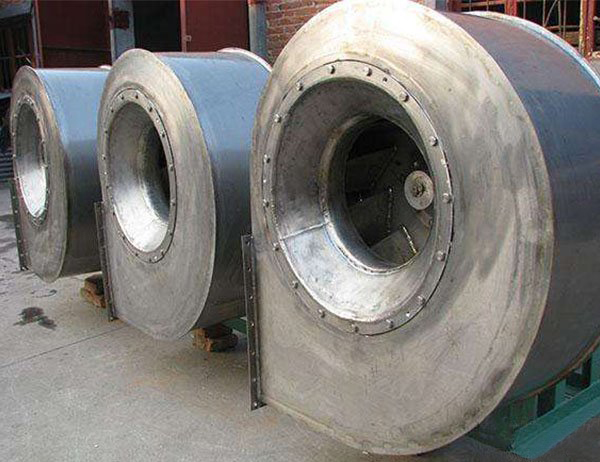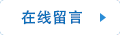Q Q：921911044

# 风机的全压是什么意思？

2019-09-16150mm,4个弯头。需保证支管末端端口处35m/s的风速。以上规格，请参考计算风机静压。

1、计算管道摩阻：R=[(λ/D)*(ν^2*γ/2)]*65

=[(0.016/0.3)*(35^2*1.2/2)]*65

=2548Pa

R1=[(λ/D)*(ν^2*γ/2)]*65

=[(0.016/0.15)*(35^2*1.2/2)]*10=784Pa

2、计算300mm弯头摩阻，设弯头为90度，且半径等于300mm,λ查表得0.23：R2=λ*(ν^2*γ/2)*2=0.23*(35^2*1.2/2)*2=338Pa

3、计算150mm弯头摩阻，设弯头为90度，且半径等于150mm,λ查表得0.23：R3=λ*(ν^2*γ/2)*4=0.23*(35^2*1.2/2)*4=676Pa3、总静压：

2548+784+338+676=4346Pa

### 联系方式：

Q Q ：921911044### 快捷链接：